×#### Thank you for registering.

One of our academic counsellors will contact you within 1 working day.

Click to Chat

1800-1023-196

+91-120-4616500

CART 0

• 0

MY CART (5)

Use Coupon: CART20 and get 20% off on all online Study Material

ITEM
DETAILS
MRP
DISCOUNT
FINAL PRICE
Total Price: Rs.

There are no items in this cart.
Continue Shopping• Complete JEE Main/Advanced Course and Test Series
• OFFERED PRICE: Rs. 15,900
• View Details

```Chapter 3: Functions – Exercise 3.3

Functions – Exercise 3.3 – Q.1

We have,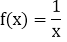Clearly, f(x) assumes real values for all real values for all x except for the values of x = 0

Hence, Domain (f) = R - {0}

We have,Clearly, f(x) assumes real values for all real values for all x except for the values of x satisfying x - 7 = 0 i.e., x = 7

Hence, Domain (f)= R - {7}

We have,We observe that f(x) is a rational function of x as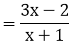is a rational expression. Clearly, f(x) assumes real values for all x except for the values of x for which x + 1 = 0 i.e., x = -1

Hence, Domain = R - {-1}

We have,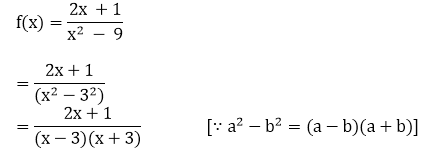We observe that f(x) is a rational function of x as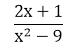is a rational expression. Clearly, f(x) assumes real values for all x except for all those values of x for which x2 - 9 = 0 i.e., x = -3, 3

Hence, Domain (f) = R - {-3, 3}.

We have,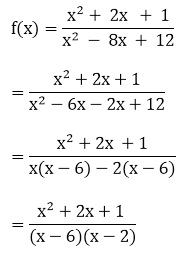Clearly, f(x) is a rational function of x as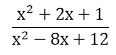is a rational expression in x. We observe that f(x) assumes real values for all x except for all those values of x for which x2 - 8x + 12 = 0 i.e., x = 2, 6

∴ Domain (f) = R - {2, 6}

Functions – Exercise 3.3 – Q.2

(i) We have,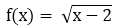Clearly, f(x) assumes real values, if

x - 2 ≥ 0

⟹ x ≥ 2

⟹ x ϵ [2, ∞)

Hence, Domain (f) = [2,∞]

(ii) We have,Clearly, f(x) assumes real values, if

x2 - 1 > 0

⟹ (x - 1)(x + 1) > 0        [∵ a2 - b2 = (a - b)(a + b)]

⟹ x < -1 or x > 1

⟹ x ϵ (-∞,-1) ∪ (1,∞)

Hence, domain (f) = (-∞, -1) ∪ (1,∞)

(iii) We have,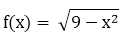Clearly, f(x) assumes real values, if

9 - x2 ≥ 0

⟹ 9 ≥ x2

⟹ x2 ≤ 9

⟹ -3 ≤ x ≤ 3

⟹ x ϵ [-3, 3]

Hence, domain (f) = [-3, 3]

(iv) We have,Clearly, f(x) assumes real values, if

x - 2 ≥ 0 and 3 - x > 0

⟹ x ≥ 2 and 3 > x

⟹ x ϵ [2, 3]

Hence, domain (f) = [2, 3).

Functions – Exercise 3.3 – Q.3

We have,We observe that f(x) is a rational function of x asis a rational expression. Clearly, f(x) assumes real values for all x except for the values of x for which

bx - a = 0 i a., bx = a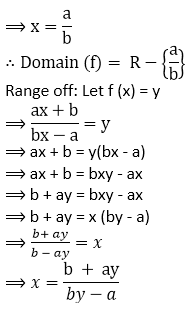Clearly, x will take real value for all x ϵ R except for

by - a = 0

⟹ by = aWe observe that f(x) is a rational function of x as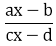is a rational expression. Clearly, f(x) assumes real values for all x except for all those values of x for which cx - d = 0 i.e., cx = d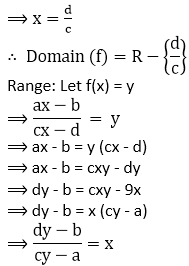Clearly, x assumes real values for all y except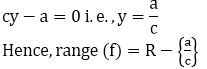We have,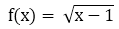Clearly, f(x) assumes real values, if

x - 1 ≥ 0

⟹ x ≥ 1

⟹ x ϵ [1, ∞)

Hence, domain(f) = [1,∞)

Range: For x ≥ 1, we have,

x - 1 ≥ 0⟹ f(x) ≥ 0

Thus, f(x) takes all real values greater than zero.

Hence, range(f) = [0, ∞)

We have,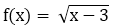Clearly, f(x) assumes values, if

x - 3 ≥ 0

⟹ x ≥ 3

⟹ x ϵ [3,∞)

Hence, domain(f) = [3,∞)

Range: For x ≥ 3,we have,

⟹ x - 3 ≥ 0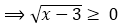⟹ f(x) ≥ 3

Thus, f(x) takes all real values greater than zero.

Hence, range(f) = [0,∞)

We have,Domain of f : Clearly, f(x) is defined for all x ϵ R except for which

2 - x ≠ 0 i.e., x ≠ 2

Hence, domain (f) = R - (2)

Range of f : Let f(x) = yWe have,

f(x)= |x - 1|

Clearly, f(x) is defined for all x ϵ R

⟹ Domain (f) = R

Range: Let f(x) = y

⟹ |x - 1|= y

⟹ f(x) ≥ 0 ∀ × ϵ R

It follows from the above relation that y takes all real values greater or equal to zero.

∴  Range (f) = [0, ∞)
```### Course Features

• 728 Video Lectures
• Revision Notes
• Previous Year Papers
• Mind Map
• Study Planner
• NCERT Solutions
• Discussion Forum
• Test paper with Video Solution